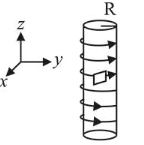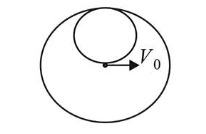# An electron gun is placed inside a long solenoid of radius R

Question:

An electron gun is placed inside a long solenoid of radius $R$ on its axis. The solenoid has $\mathrm{n}$ turns/length and carries a current I. The electron gun shoots an electron along the radius of the solenoid with speed $v$. If the electron does not hit

the surface of the solenoid, maximum possible value of $v$ is (all symbols have their standard meaning):1. (1) $\frac{e \mu_{0} n \mathrm{IR}}{m}$

2. (2) $\frac{e \mu_{0} n \mathrm{IR}}{2 m}$

3. (3) $\frac{e \mu_{0} n \mathrm{IR}}{4 m}$

4. (4) $\frac{2 e \mu_{0} n \mathrm{IR}}{m}$

Correct Option: , 2

Solution:

(2) Magnetic field inside the solenoid is given by

$B=\mu_{0} n I$

Here, $n=$ number of turns per unit lengthThe path of charge particle is circular. The maximum

possible radius of electron $=\frac{R}{2}$

$\therefore \quad \frac{m V_{\max }}{q B}=\frac{R}{2}$

$\Rightarrow V_{\max }=\frac{q B R}{2 m}=\frac{e R \mu_{0} n I}{2 m}$